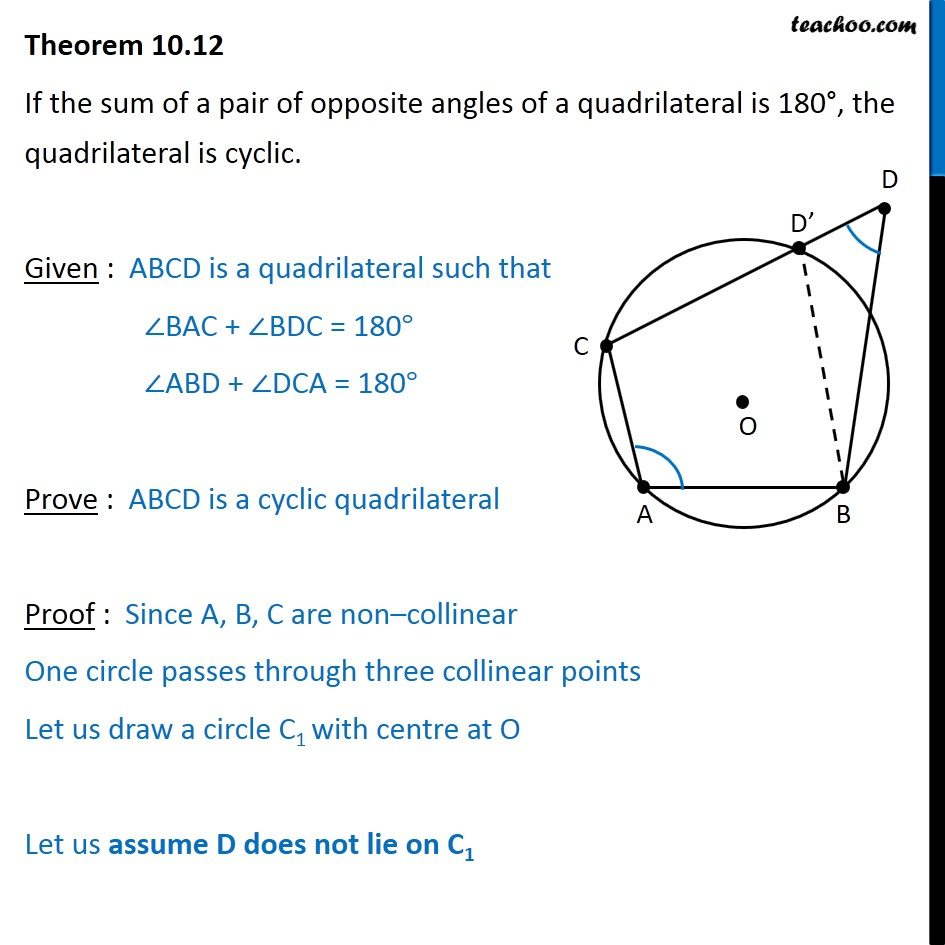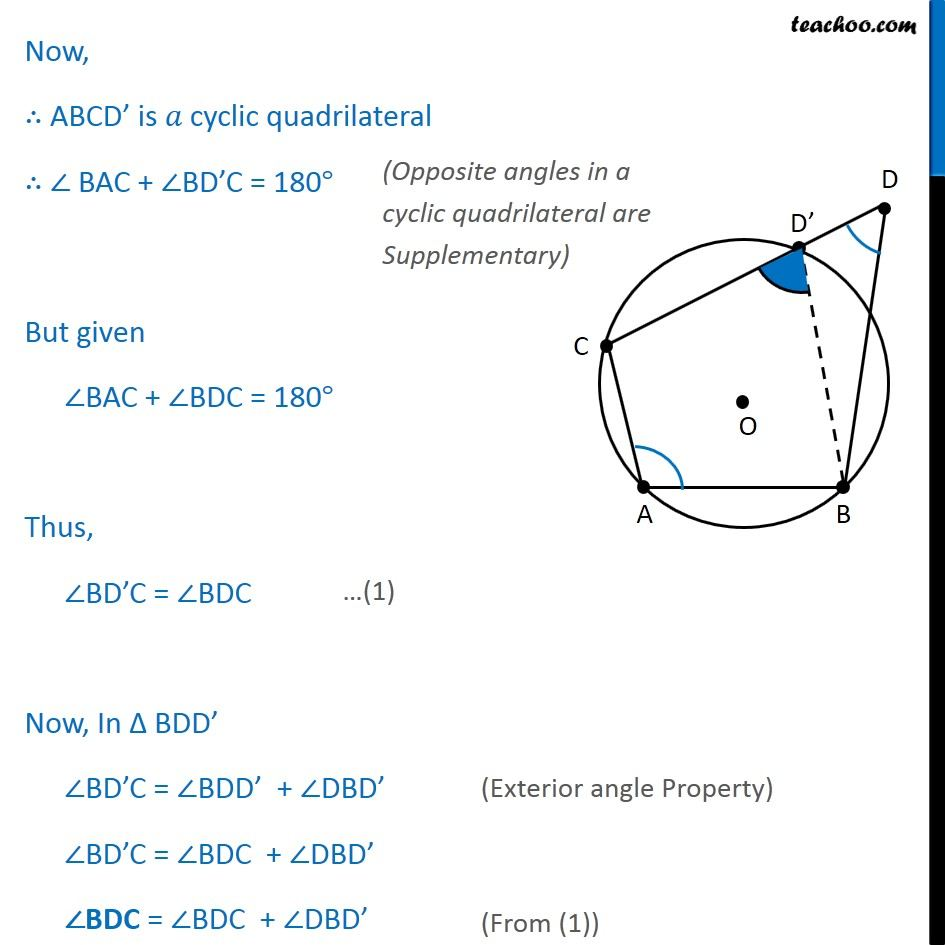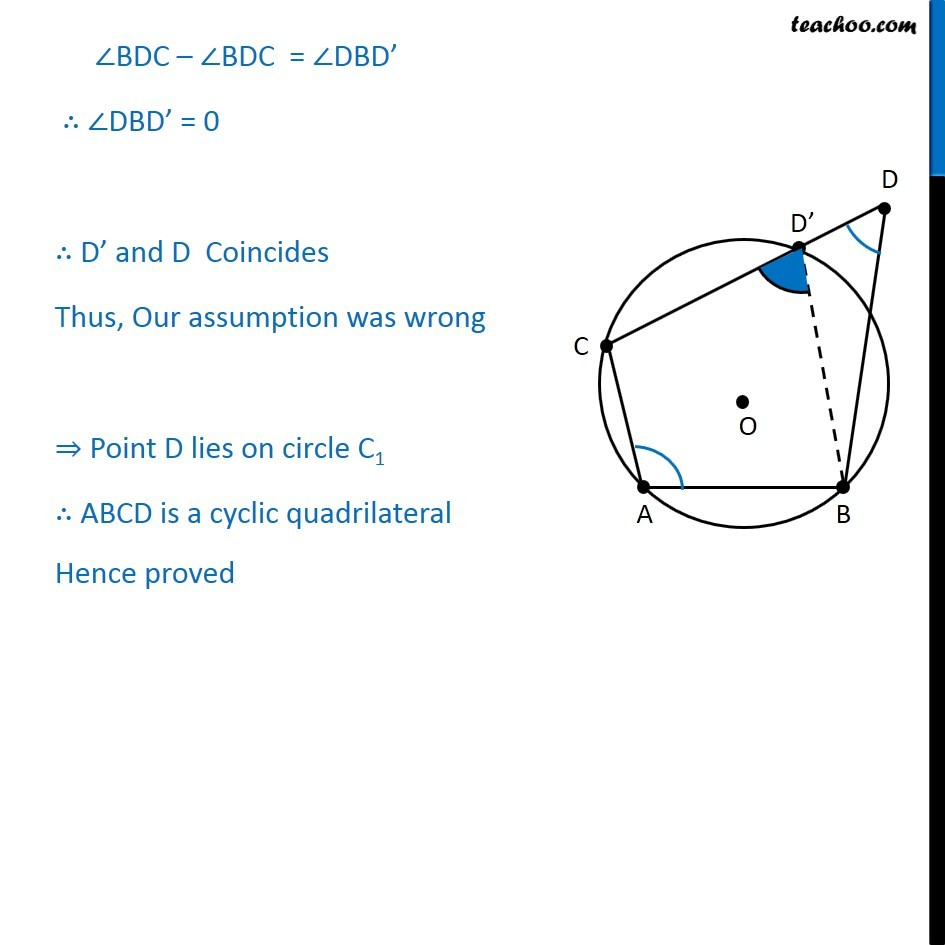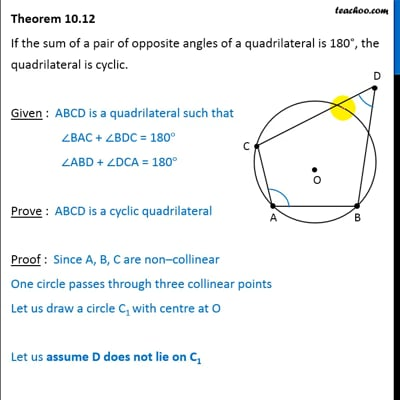Theorems

Chapter 10 Class 9 Circles
Serial order wiseThis video is only available for Teachoo black users

Get live Maths 1-on-1 Classs - Class 6 to 12

### Transcript

Theorem 10.12 If the sum of a pair of opposite angles of a quadrilateral is 180 , the quadrilateral is cyclic. Given : ABCD is quadrilateral such that BAC + BDC = 180 Prove : ABCD is a cyclic quadrilateral Proof : Since A, B, C are non collinear One circle passes through three collinear points Let us draw a circle C1 with centre at O Let us assume D does not lie on C1 Now, ABCD is cyclic quadrilateral BAC + BD C = 180 But given BAC + BDC = 180 Thus, BD C = BDC Now, In BDD BD C = BDD + DBD BD C = BDC + DBD BDC = BDC + DBD BDC BDC = DBD DBD = 0 D and D Coincides Our assumption was wrong Point D lies on circle C1 A, B, C, D are concyclic. Hence proved